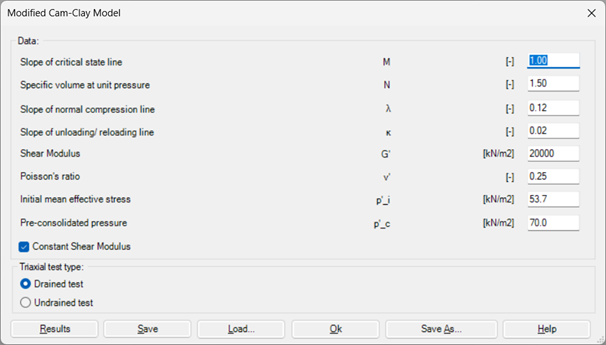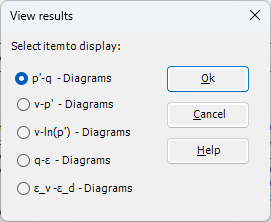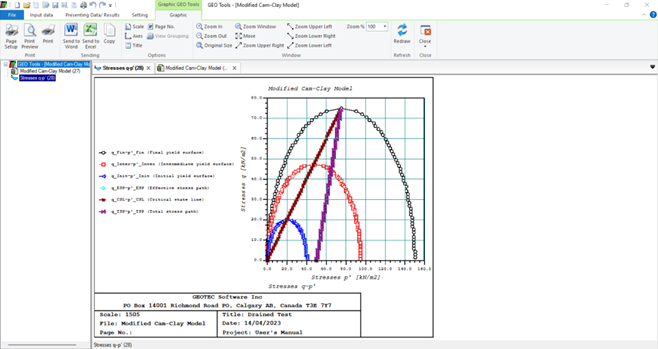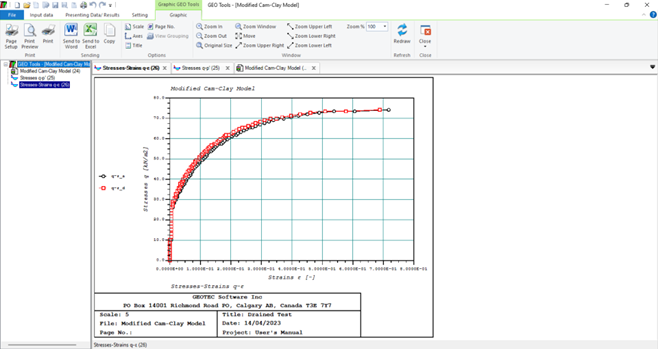# Analysis of Shallow Foundations

Shallow foundation problems that can be analyzed are, Figure 2:
1- Stresses in soil
2- Strains in soil
3- Displacements in soil
4- Consolidation settlement
5- Degree of consolidation
6- Time-settlement curve
7- Displacement of rigid raft
8- Consolidation of rigid raft
9- Settlement of footing groups
10- Analysis of a beam foundation
11- Modified Cam-Clay Model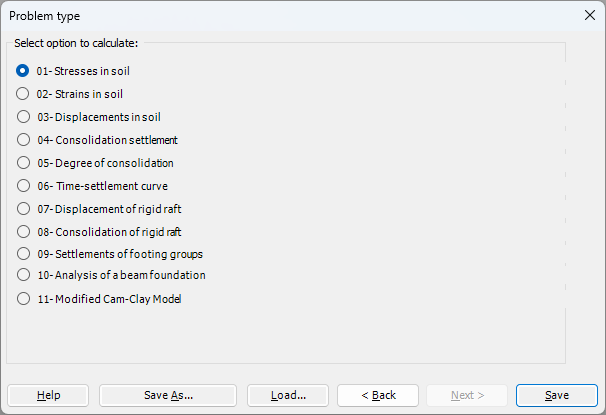Figure 2 Menu "Shallow foundation problems"
Stresses in the soil can be determined and presented under any point on the foundation or at any plane under the foundation, Figure 3 and Figure 4.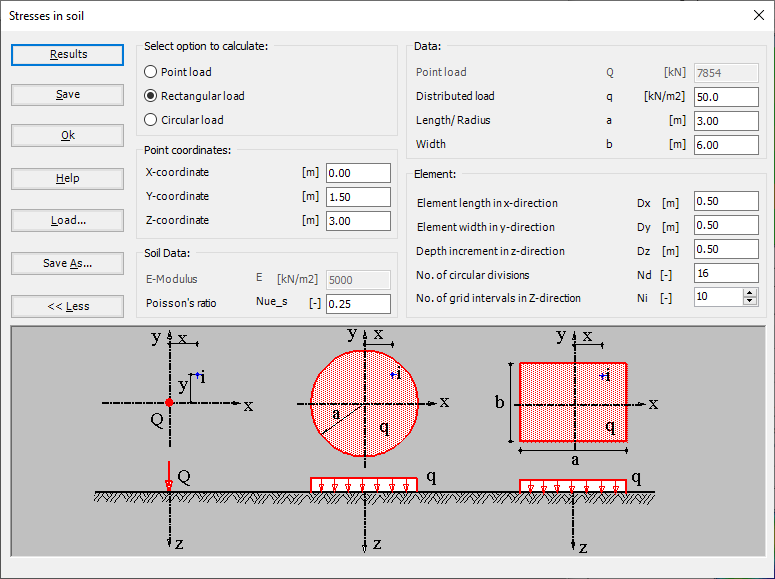Figure 3 "Stress in soil"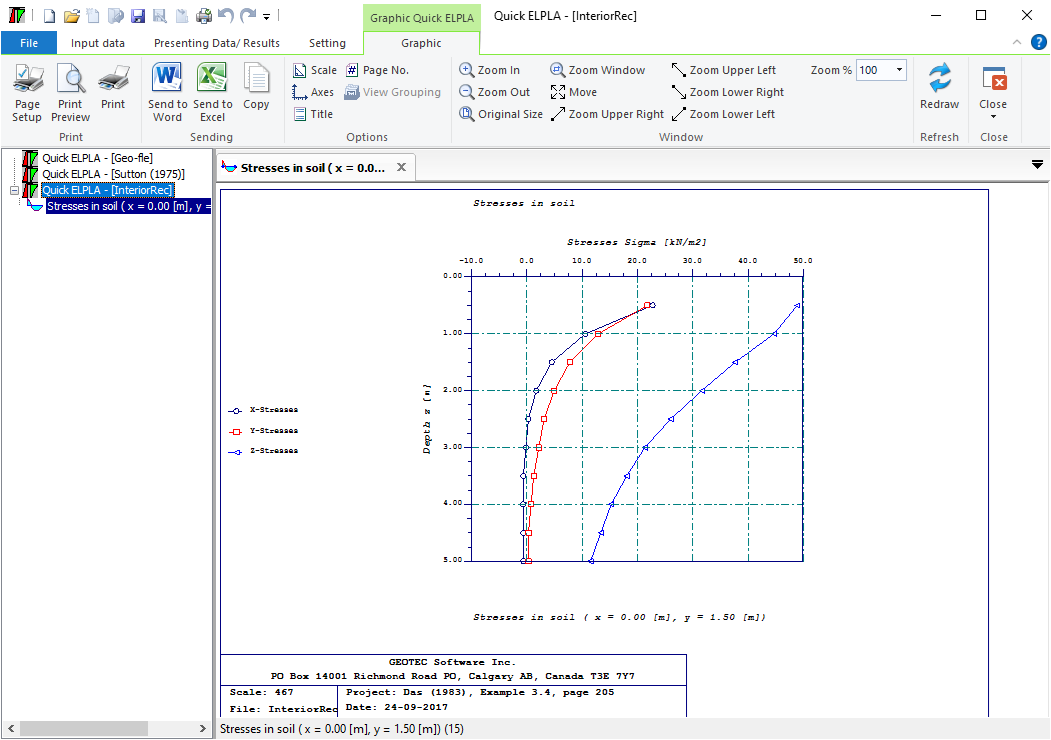Figure 4 Results as diagrams under a point on the foundation
Strains in the soil can be determined and presented under any point on the foundation or at any plane under the foundation (Figure 5 and Figure 6).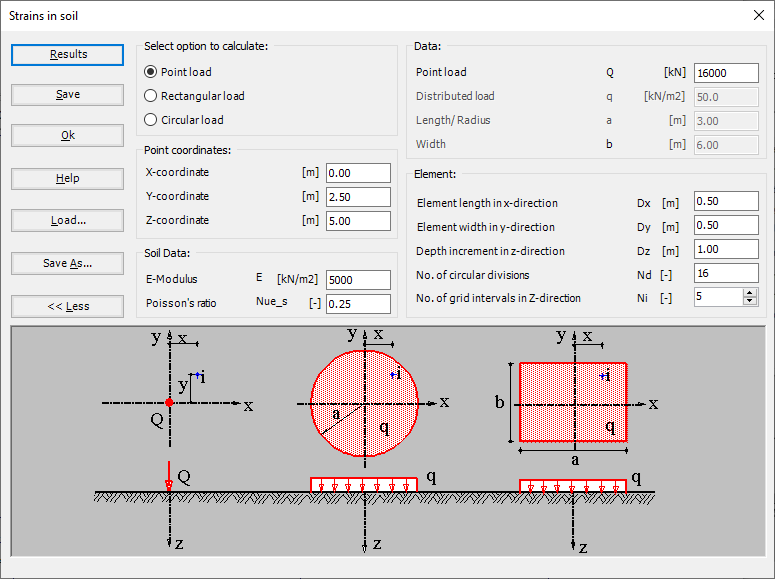Figure 5 "Strain in soil"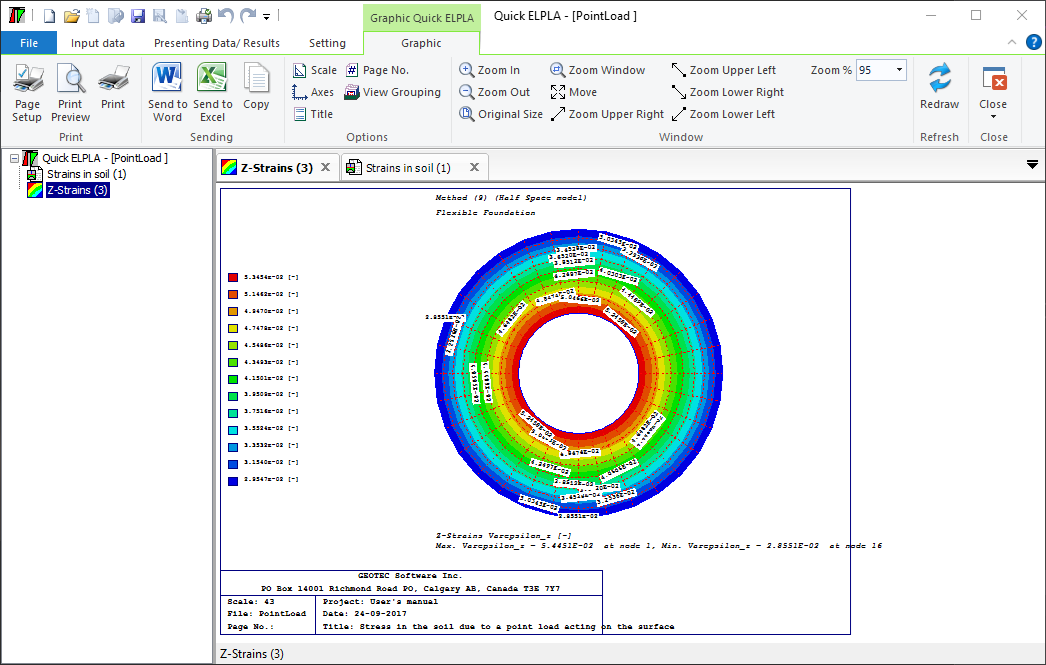Figure 6 Strains in the soil as contour
Displacements in the soil can be determined and presented under any point on the foundation or at any plane under the foundation (Figure 7 and Figure 8).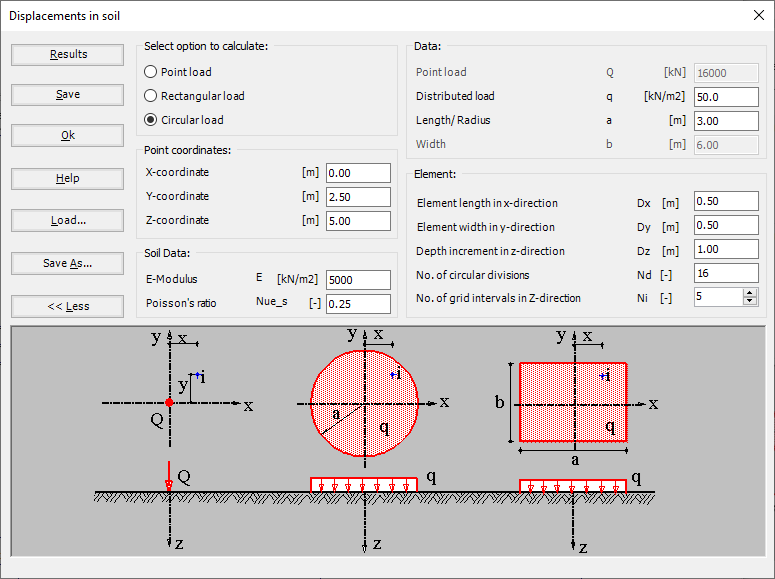Figure 7 "Displacements in the soil"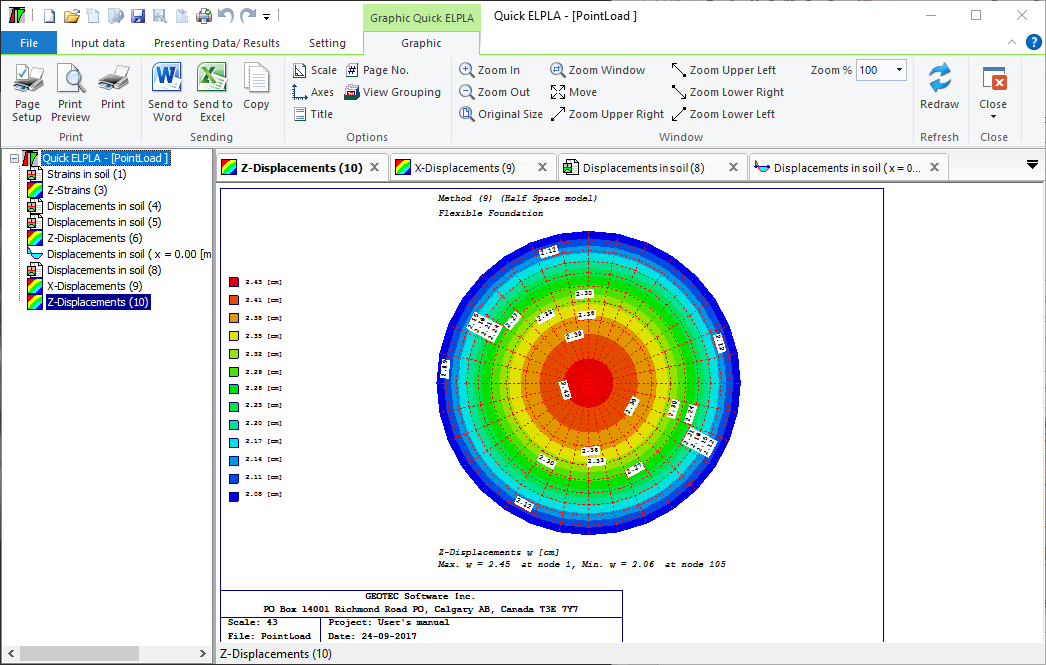Figure 8 Displacements in the soil as contour
Final consolidation settlement for any irregular foundation on layered soil can be determined, Figures 9 to ‎11.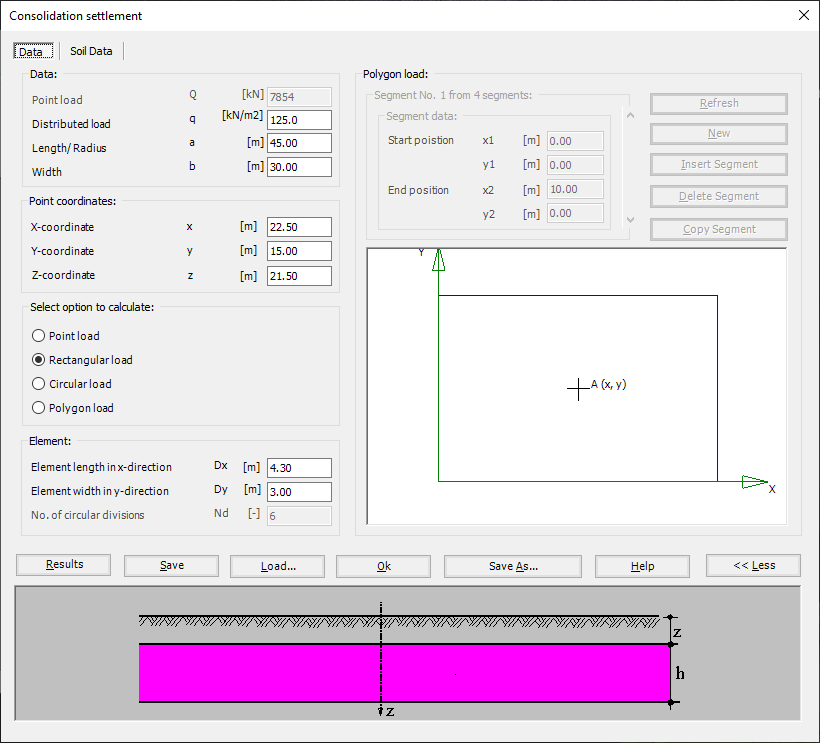Figure 9 "Consolidation settlement"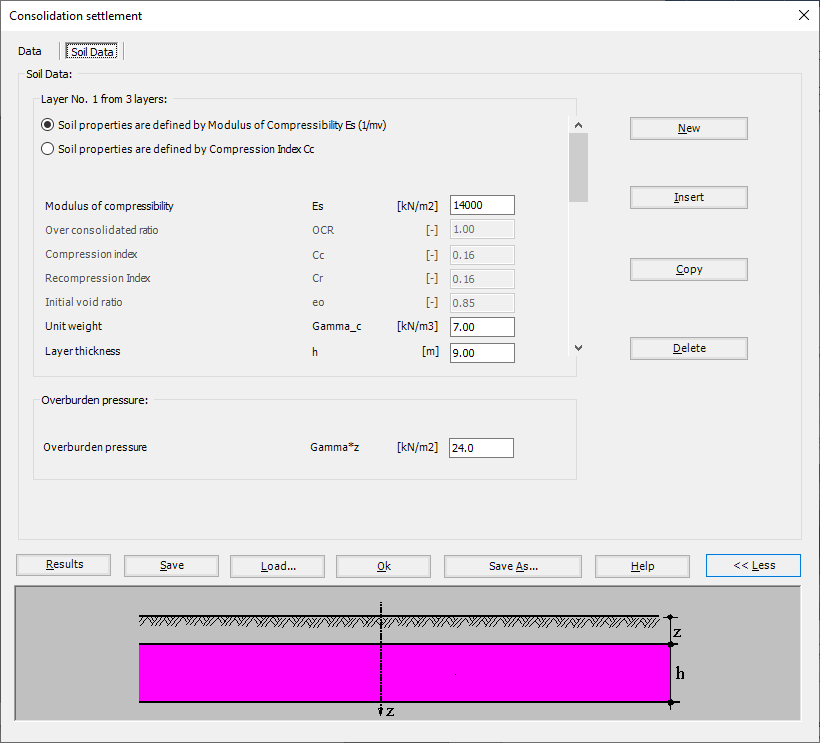Figure 10 "Consolidation settlement", Soil data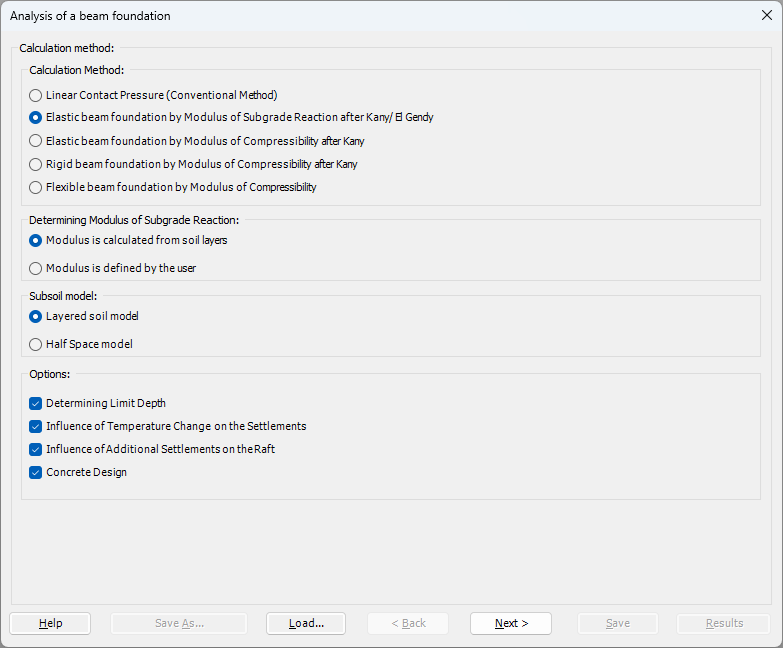Figure 11 Final consolidation settlement under a rectangular loaded area
Degree of consolidation The degree of consolidation for linear and nonlinear analysis can be determined for multi-layered clayey soil with different calculation methods. Cyclic loading for more than 14 types of cyclic loading can be also determined. Initial pore water pressure on the clay layers can be defined by the user or determined due to different types of load geometries on the surface. The consolidation settlement in the place (xy) due to point load, rectangular uniform load, or circular uniform load on the surface can be determined (Figure 12 to Figure 16).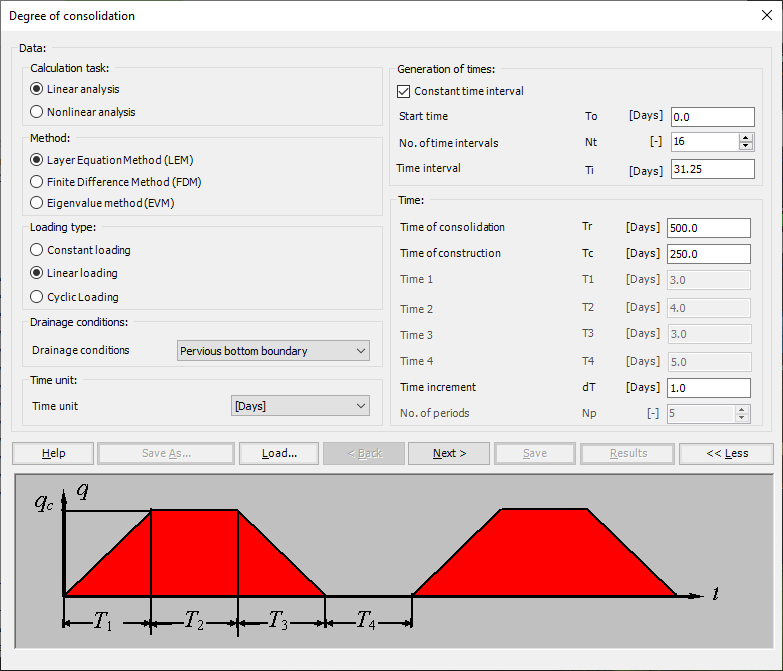Figure 12 "Degree of consolidation"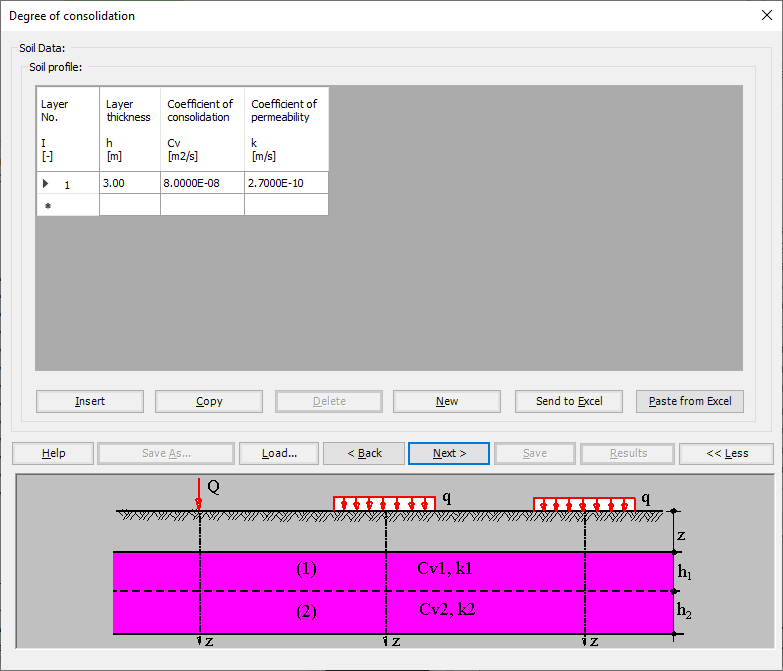Figure 13 Degree of consolidation", Soil data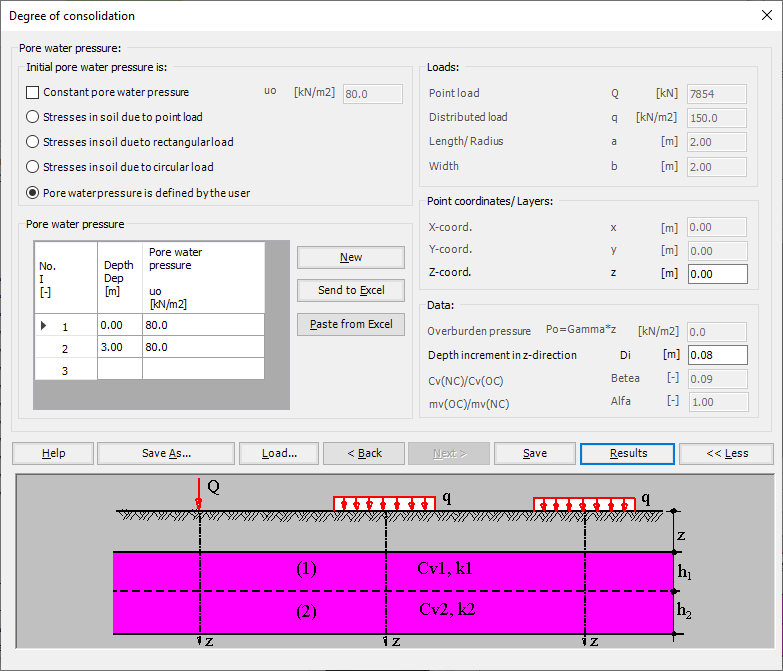Figure 14 "Degree of consolidation", Pore water pressure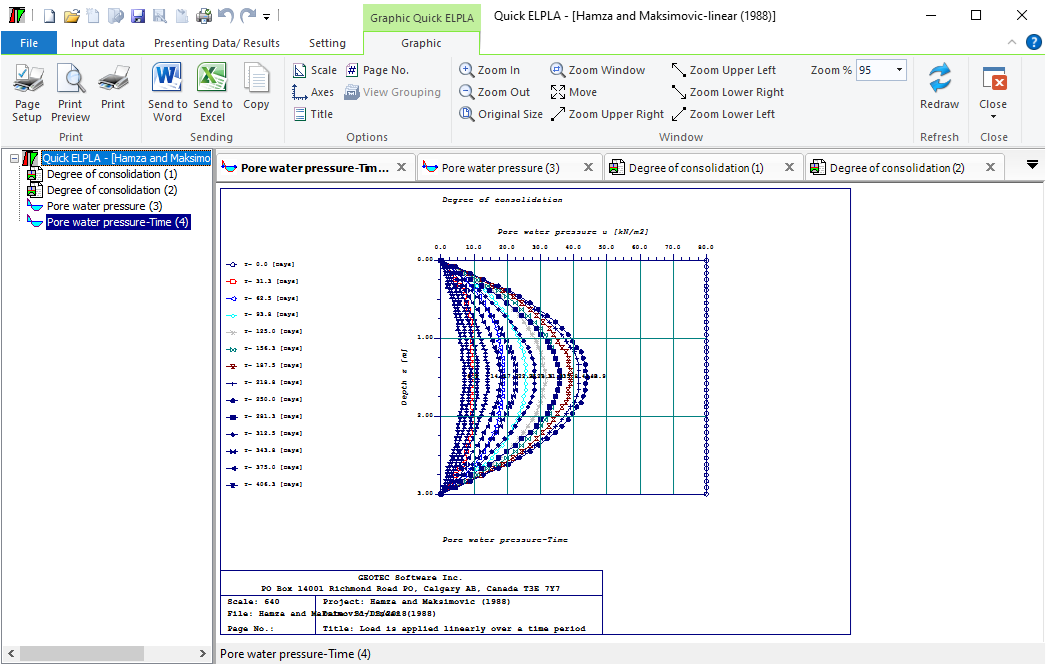Figure 15 Pore water pressure with time for multi-layered clayey soil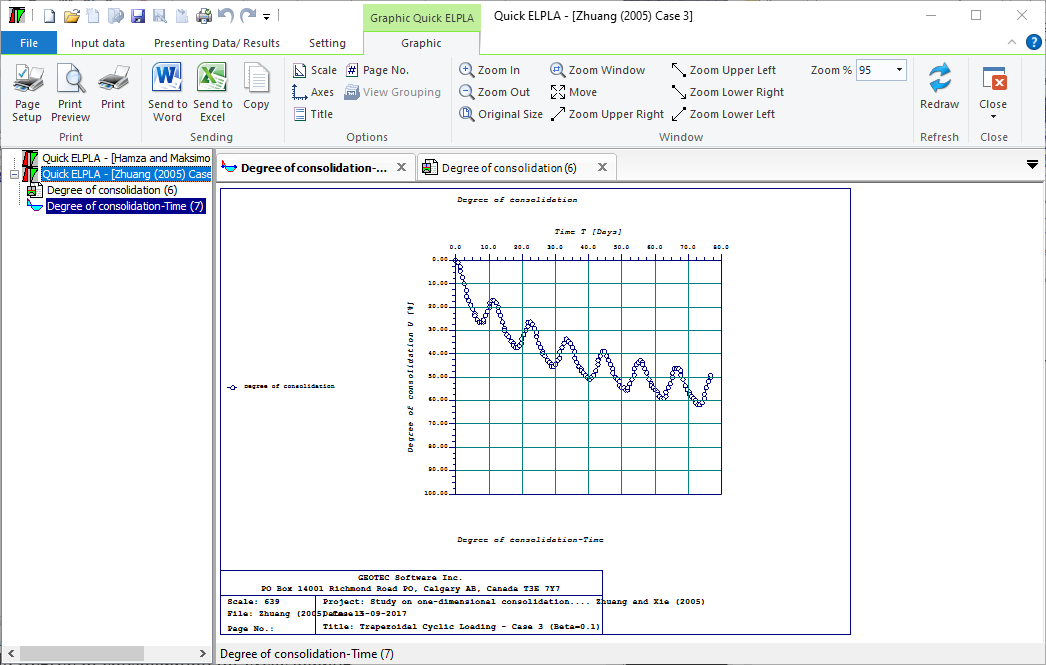The time-settlement curve of a building can be determined by three methods. Also, settlements during the construction time and the final settlement from measurement results can be estimated through extrapolation. The load can be applied in steps with time, (Figure 17 to Figure 20).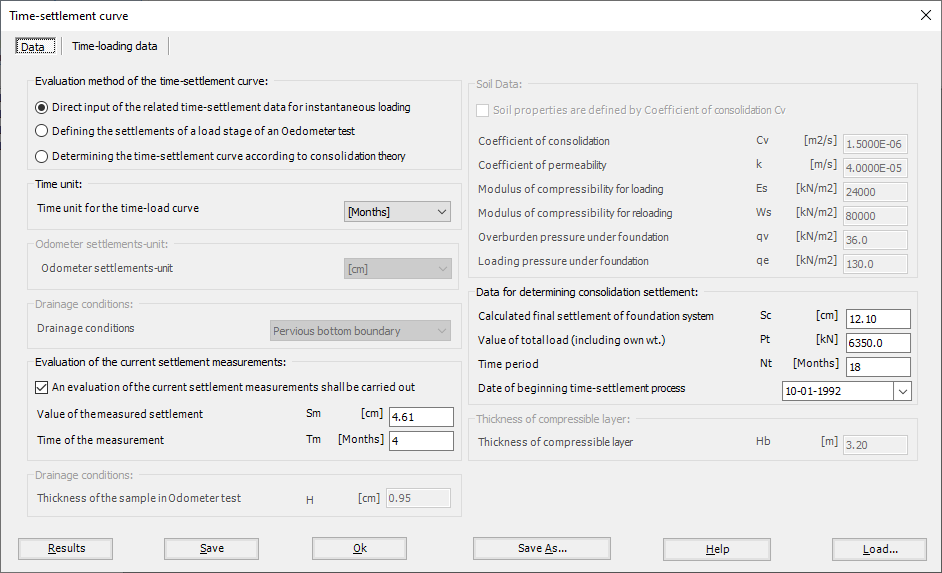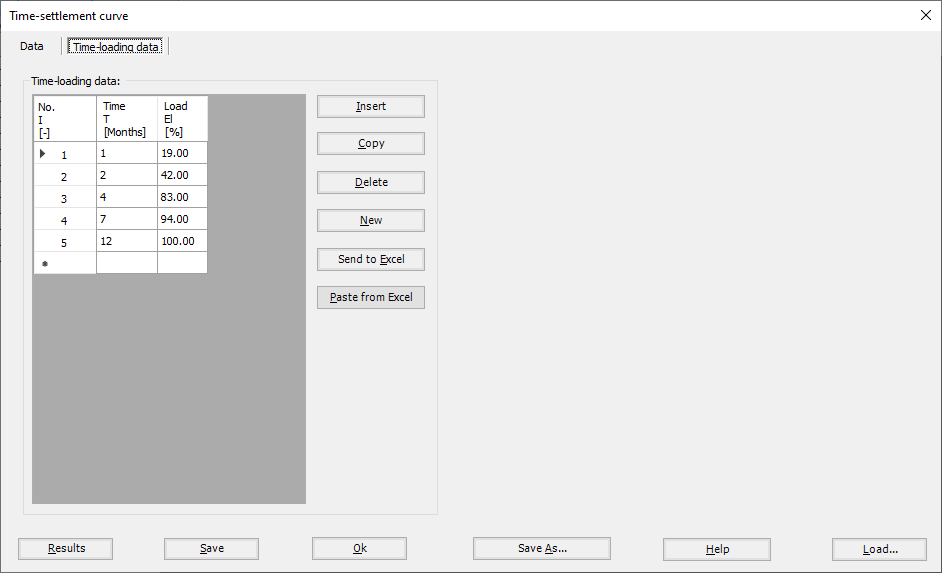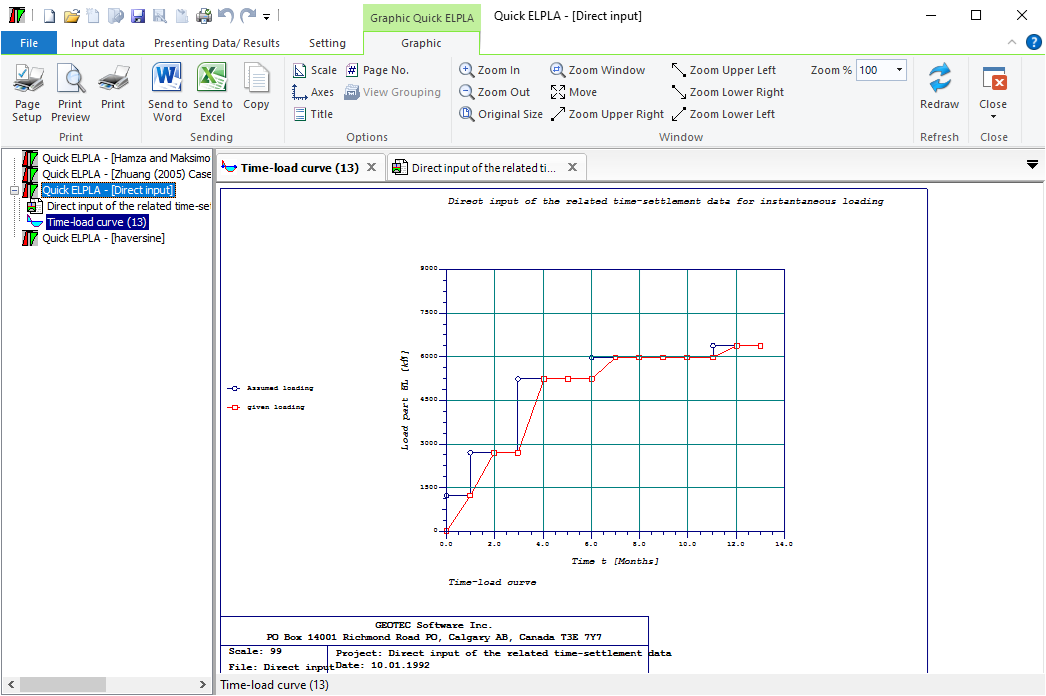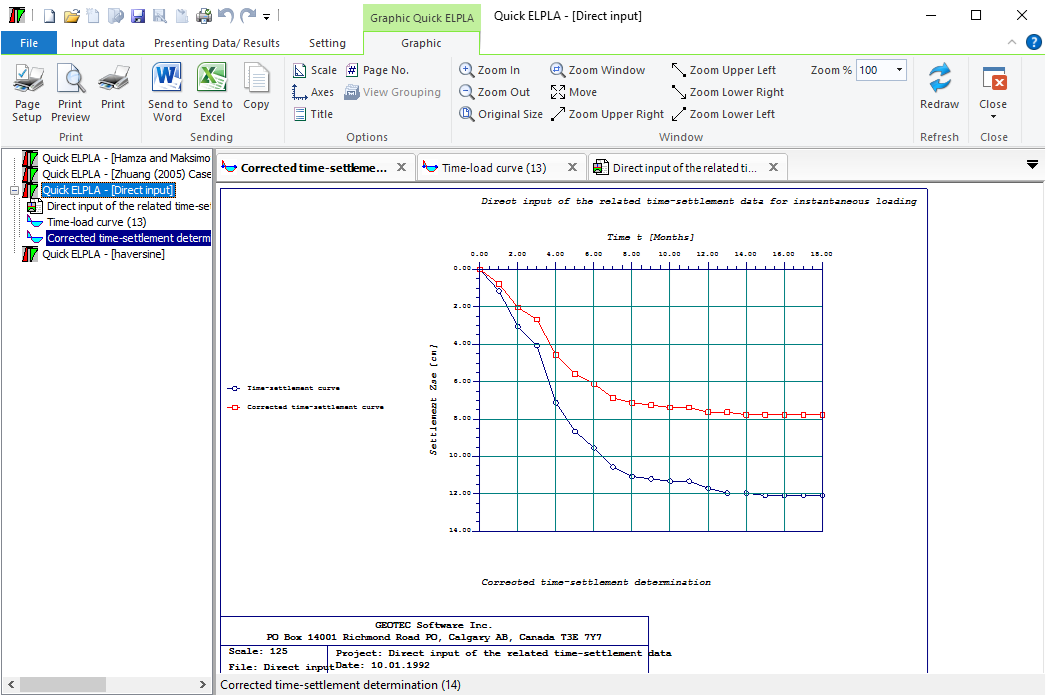Figure 20 Corrected time-settlement determination
Displacement and contact pressure under a rigid rectangular or circular raft on a soil layer can be determined analytically or numerically. Also, half-space soil model can be considered, Figure 21 and Figure 22.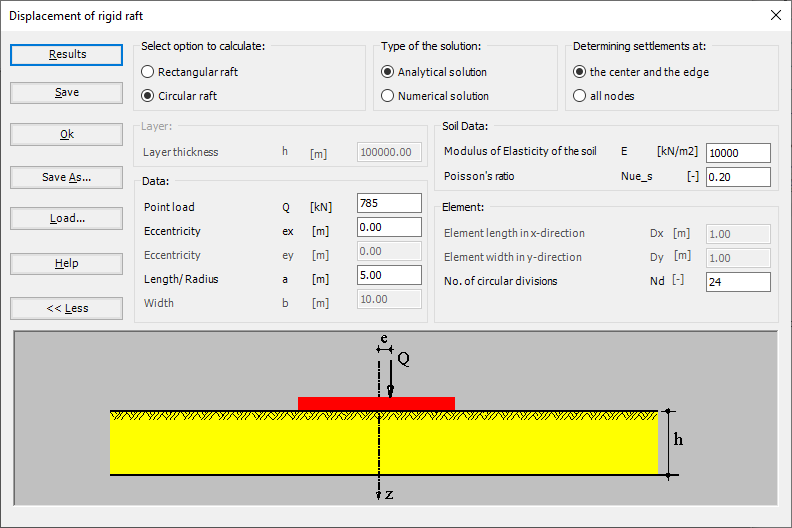Figure 21 "Displacement of rigid raft"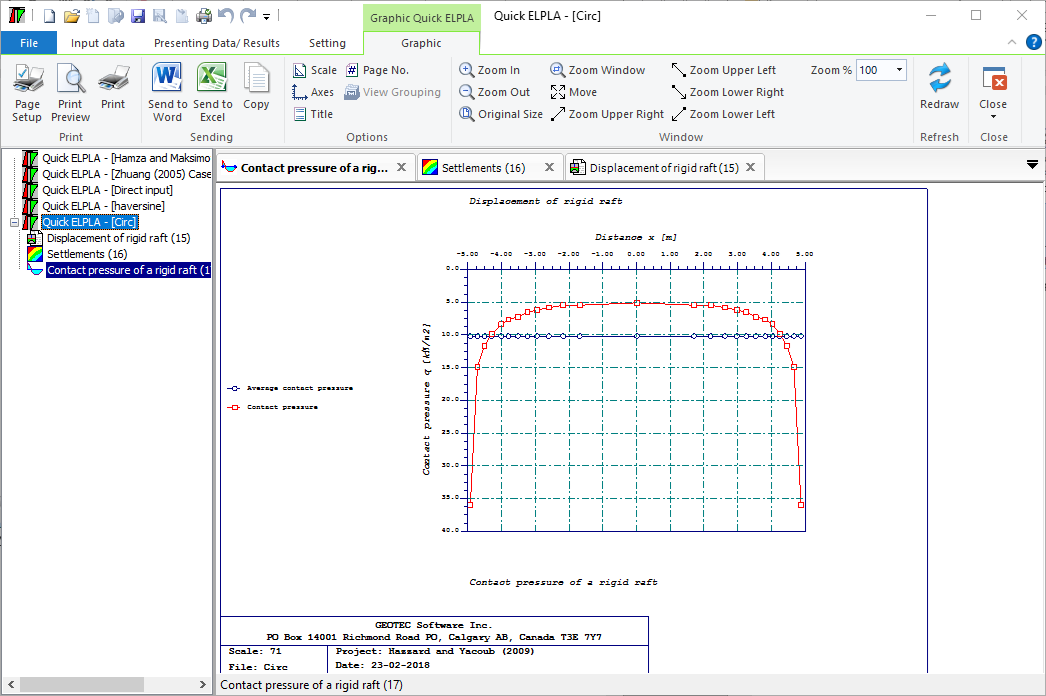Figure 22 Contact pressure of a rigid raft
Final consolidation settlement and contact pressure under a rigid rectangular or circular raft on a clay layer can be determined analytically or numerically. Also, a half-space soil model can be considered, (Figure 23 to Figure 25).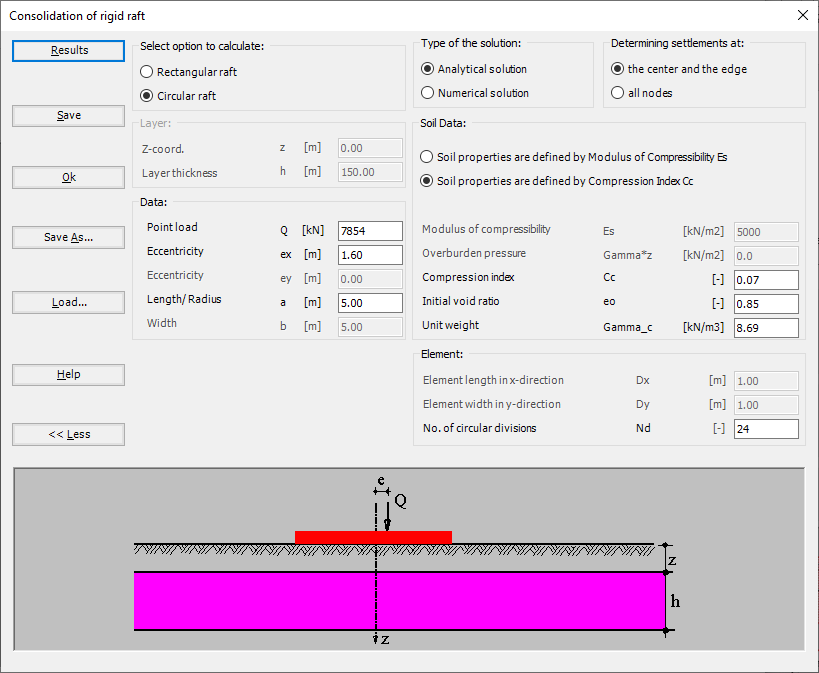Figure 23 "Consolidation of rigid raft"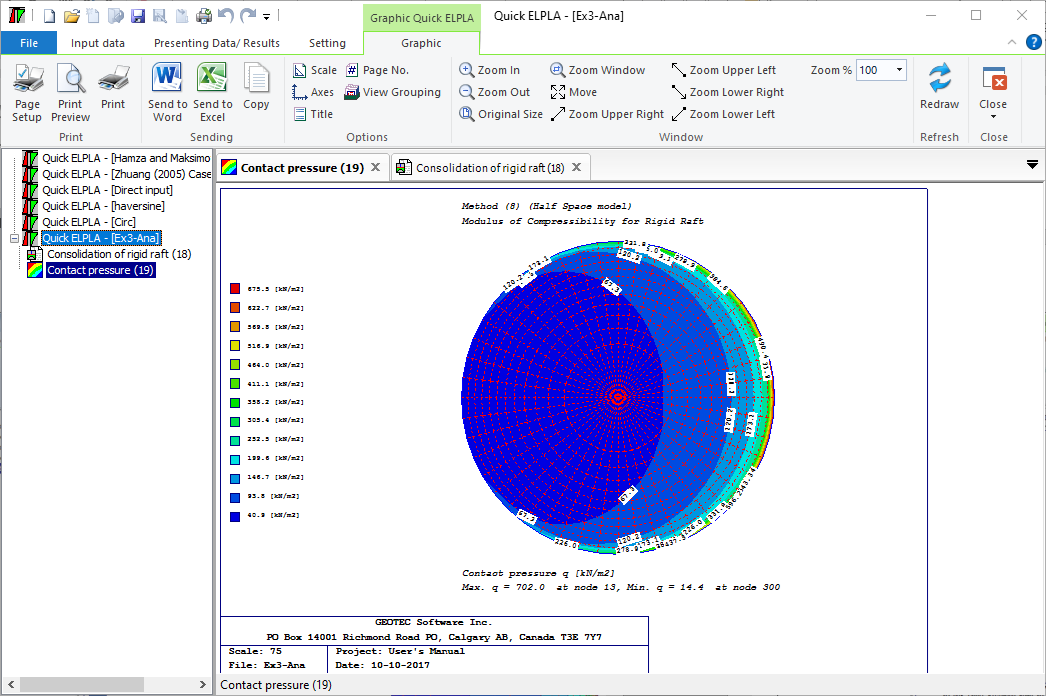Figure 24 Contact pressure as contour lines under a rigid raft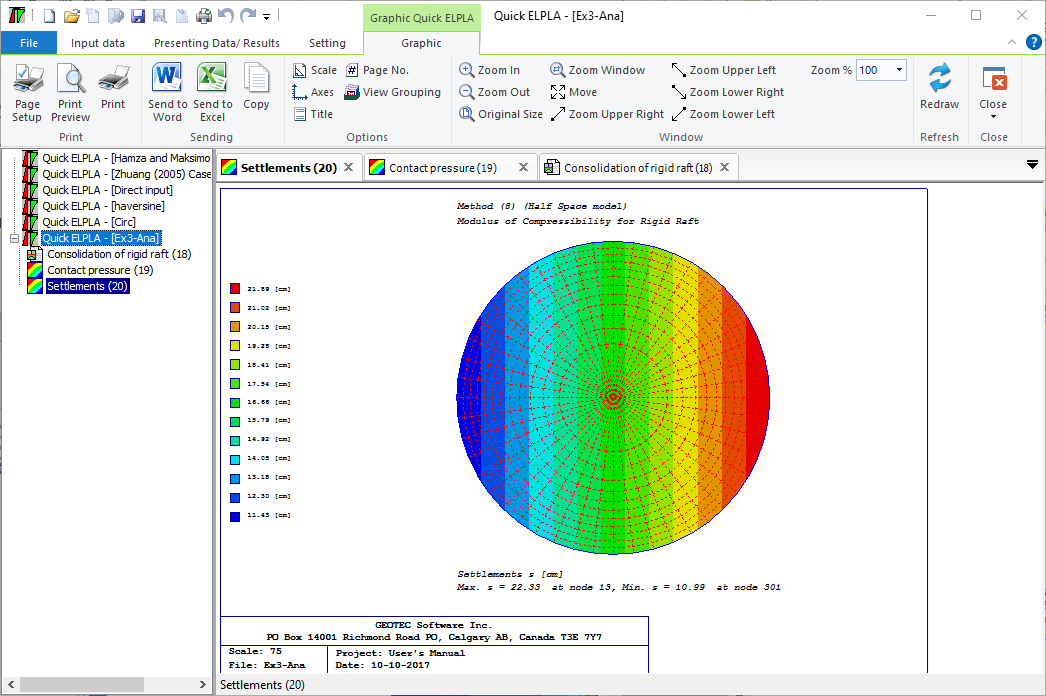Figure 25 Consolidation settlement as contour lines under a rigid raft
Limit depth and settlement of footing group on a layered soil can be determined, Figure 26 to Figure 31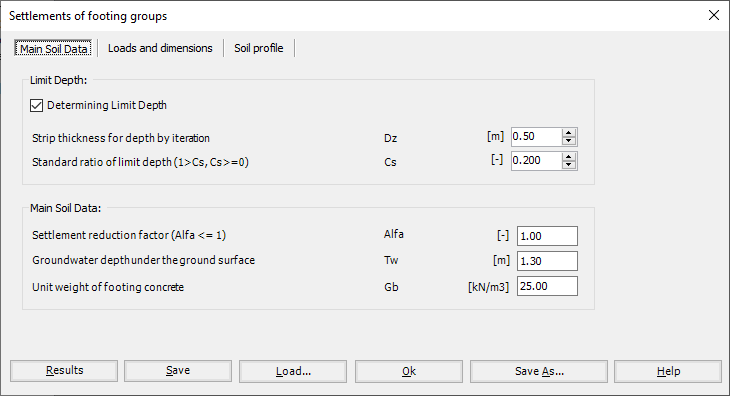Figure 26 "Settlement of footing groups"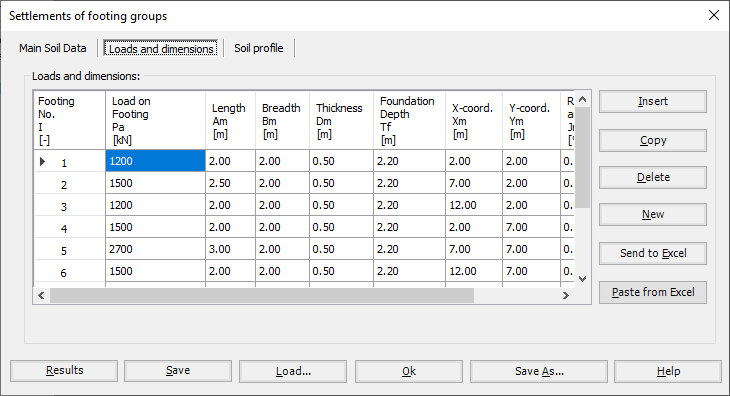Figure 27 "Settlement of footing groups", Loads and dimensions"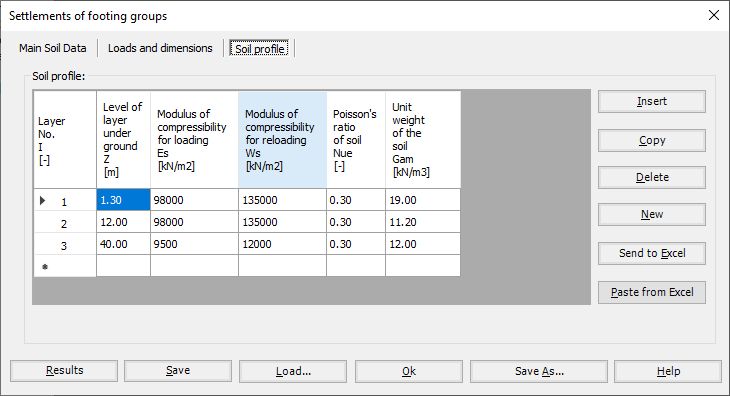Figure 28 "Settlement of footing groups", Soil profile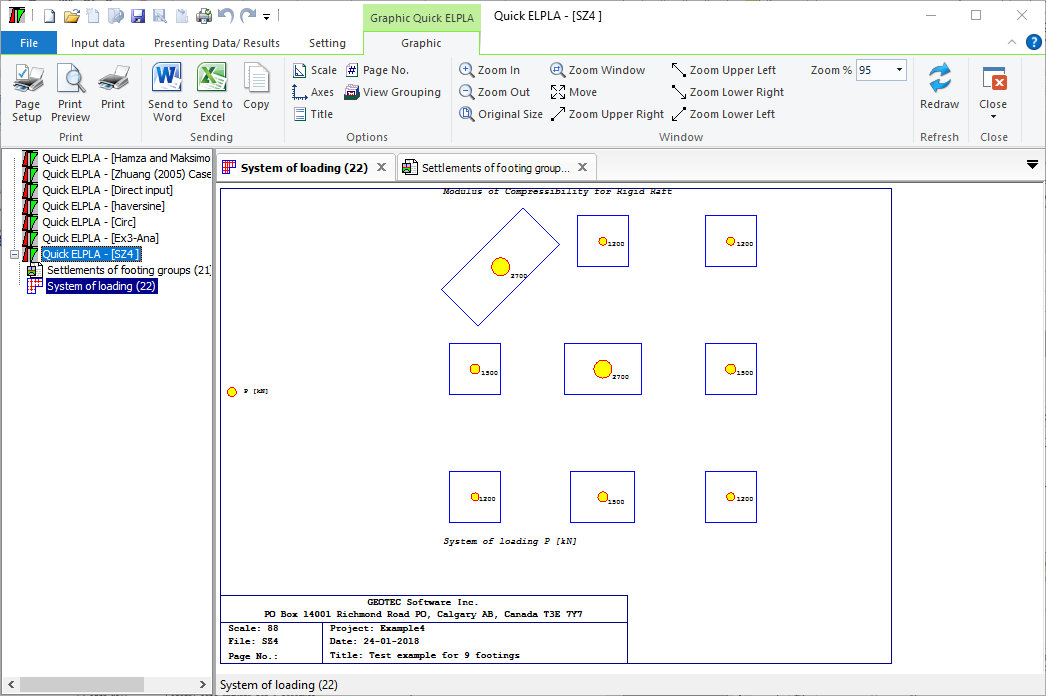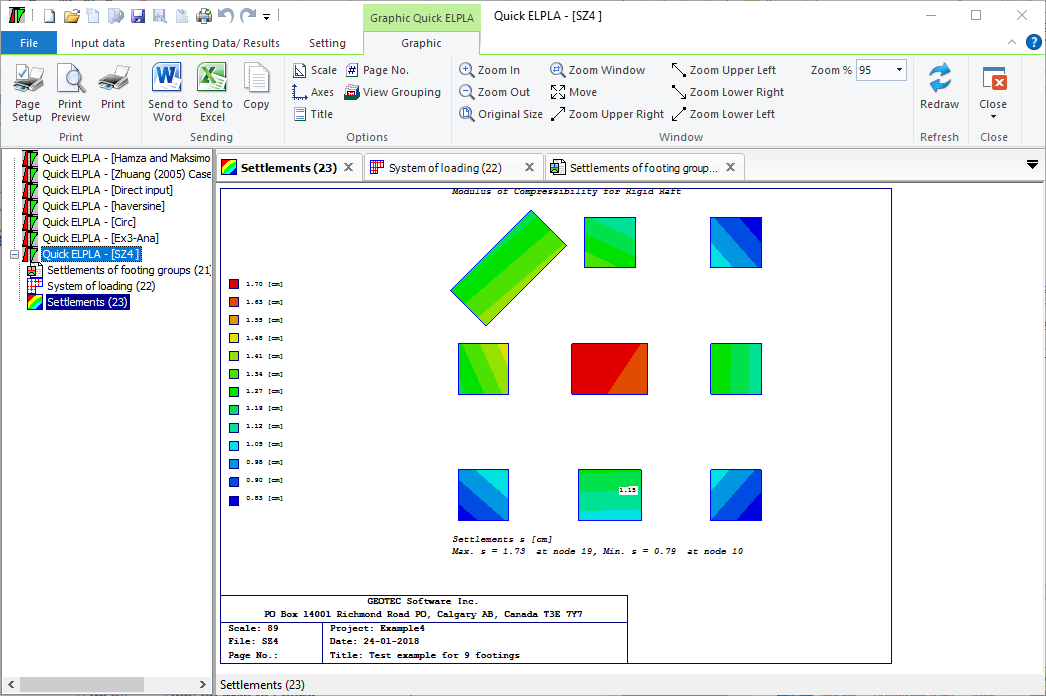Figure 30 Settlement of a group of footings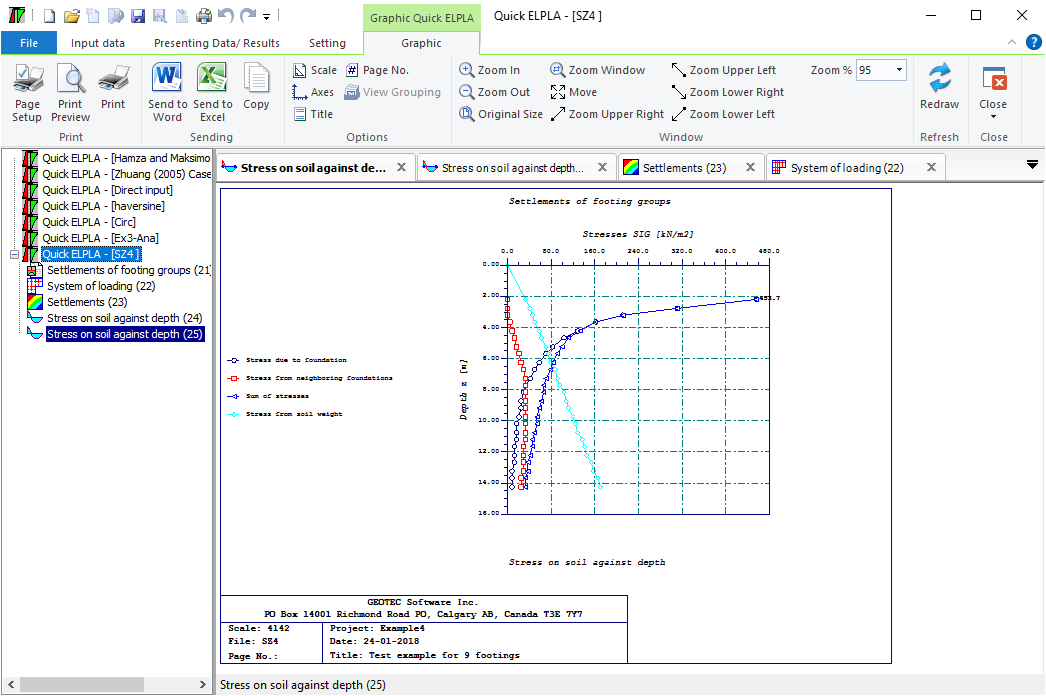Figure 31 Limit depth calculation and stress under the footing

It is possible to use the same data for analyzing beam foundations by five different conventional and refined calculation methods based on the three standard subsoil models using GEO Tools program. The subsoil models for analyzing beam foundations (standard models) available in GEO Tools are:

a) Simple assumption model

b) Winkler's model

c) Continuum model

The simple assumption model does not consider the interaction between the beam foundation and soil. The model assumes a linear distribution of contact pressures beneath the foundation. Winkler's model is the oldest and simplest one. That considers the interaction between the beam foundation and the soil. The model represents the soil as elastic springs. The continuum model is a complicated one. Also, this model considers the interaction between the beam foundation and soil. It represents the soil as a layered continuum medium.

The three standard soil models are described through five different numerical calculation methods. The methods graduate from the simplest to the more complicated one covering the analysis of the most common beam foundation problems.

According to the three standard soil models (simple assumption model - Winkler's model - Continuum model), five numerical calculation methods are considered to analyze the beam foundation as follows:

1- Linear Contact Pressure

(Simple assumption model)

2- Elastic Beam Foundation using Modulus of Subgrade Reaction by Kany/ El Gendy (1995) (Winkler's model)

3- Elastic Beam Foundation using Modulus of Compressibility by Kany (1974)

(Continuum model)

4- Rigid Beam Foundation using Modulus of Compressibility by Kany (1972)

(Continuum model)

5- Flexible Beam Foundation using Modulus of Compressibility

(Continuum model)

It is also possible to consider irregular soil layers and the thickness of the base beam that varies in each element. Furthermore, the influence of temperature changes, the additional settlement on the beam foundation, and the concrete design can be considered.

From the "Analysis of a beam foundation" command, a new form will appear with different tabs. The first tab in this form is the "Calculation Method" tab, Figure 28. You can choose the soil model. Also, you have two options to determine the modulus of the subgrade reaction, which could be calculated from soil layers or defied by the user. If you choose to calculate the modulus of the subgrade reaction from soil layers, you can define the subsoil model as a layered or half-space model. In addition, determining limit depth, the influence of temperature change on the settlements, the influence of additional settlements on the raft, and concrete design options could be selected.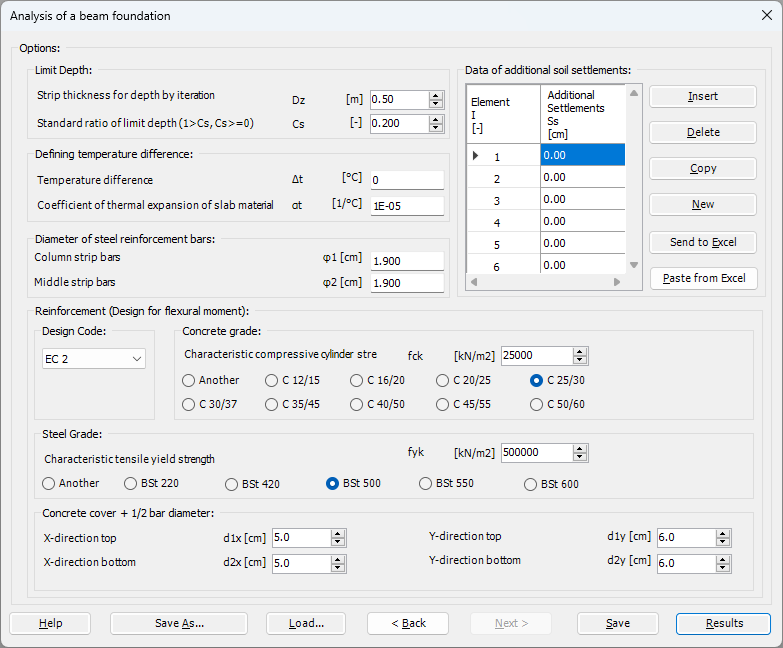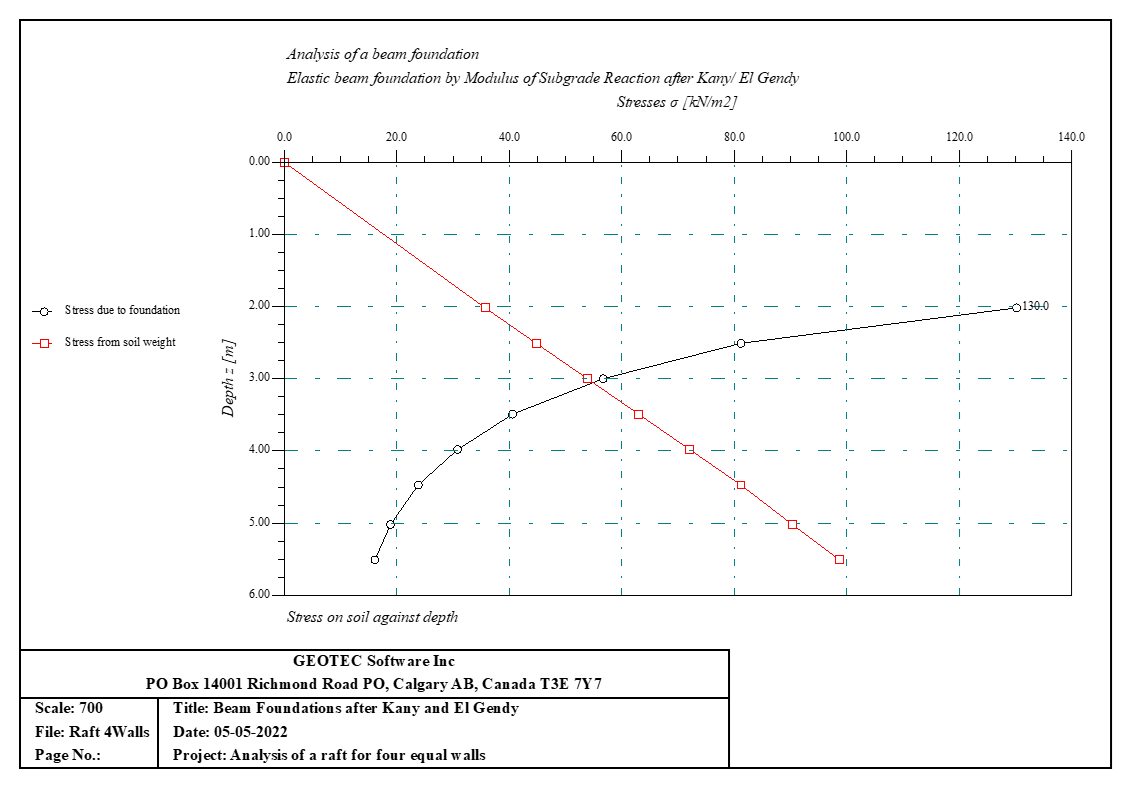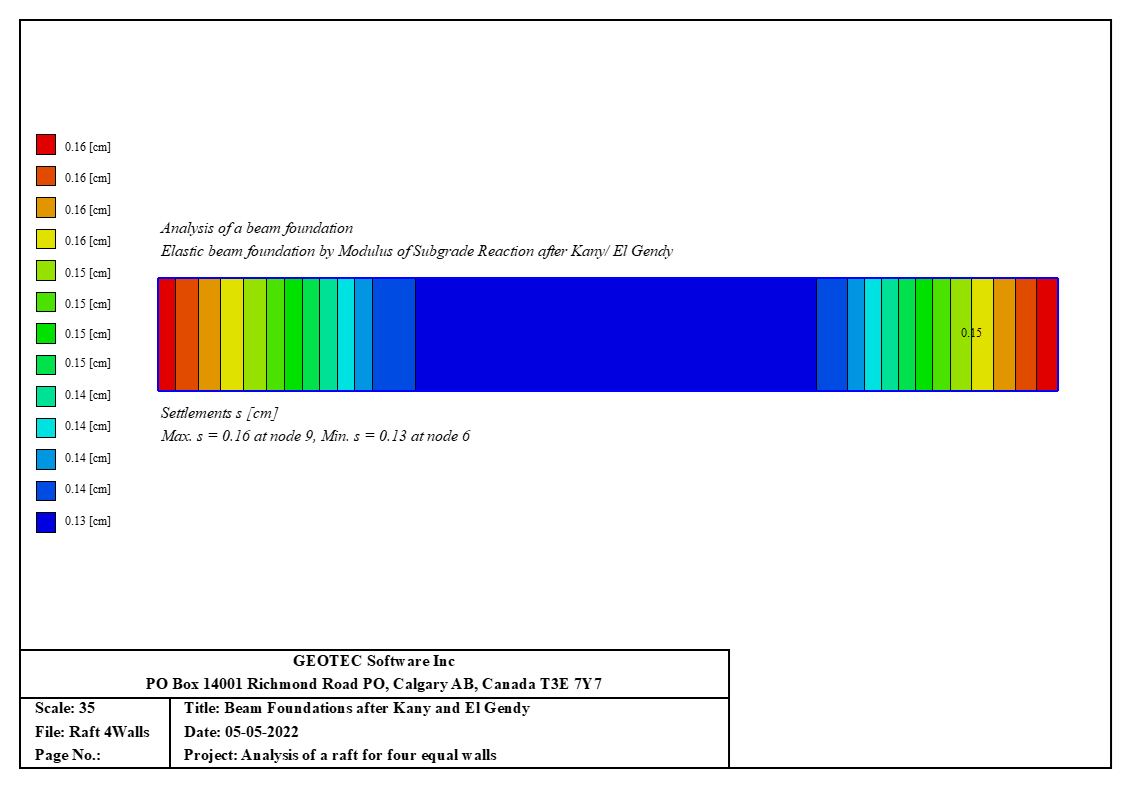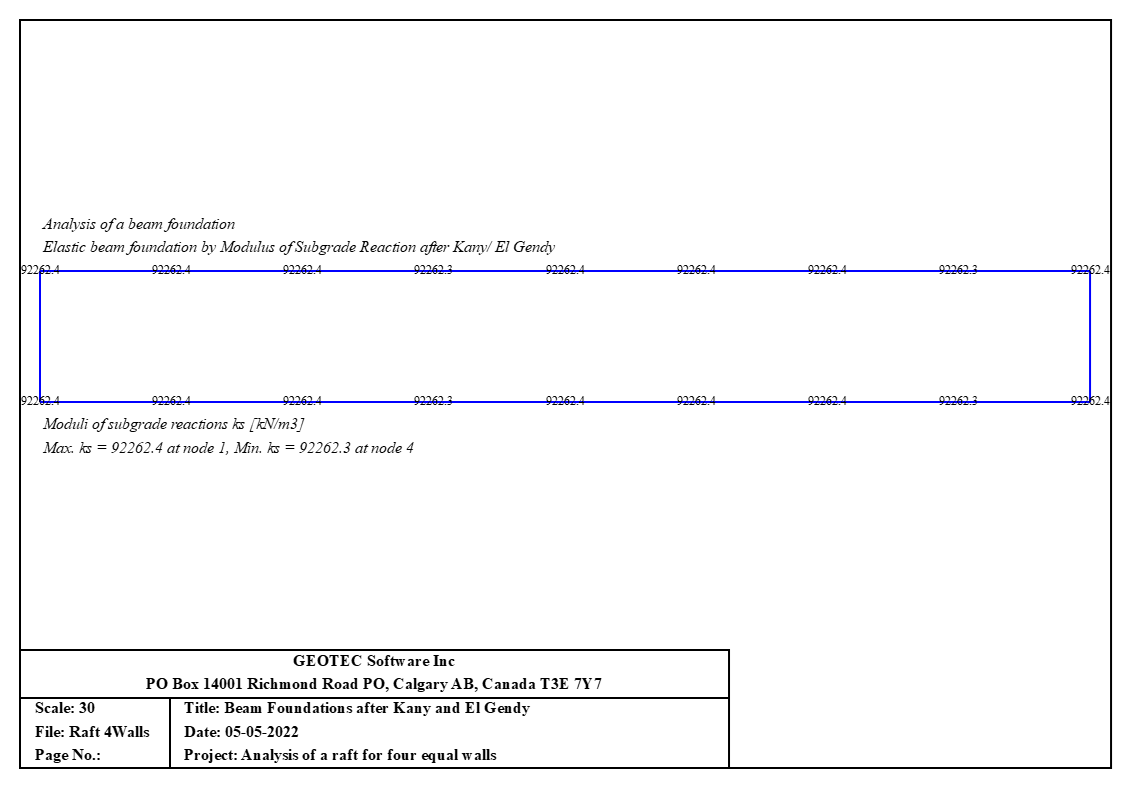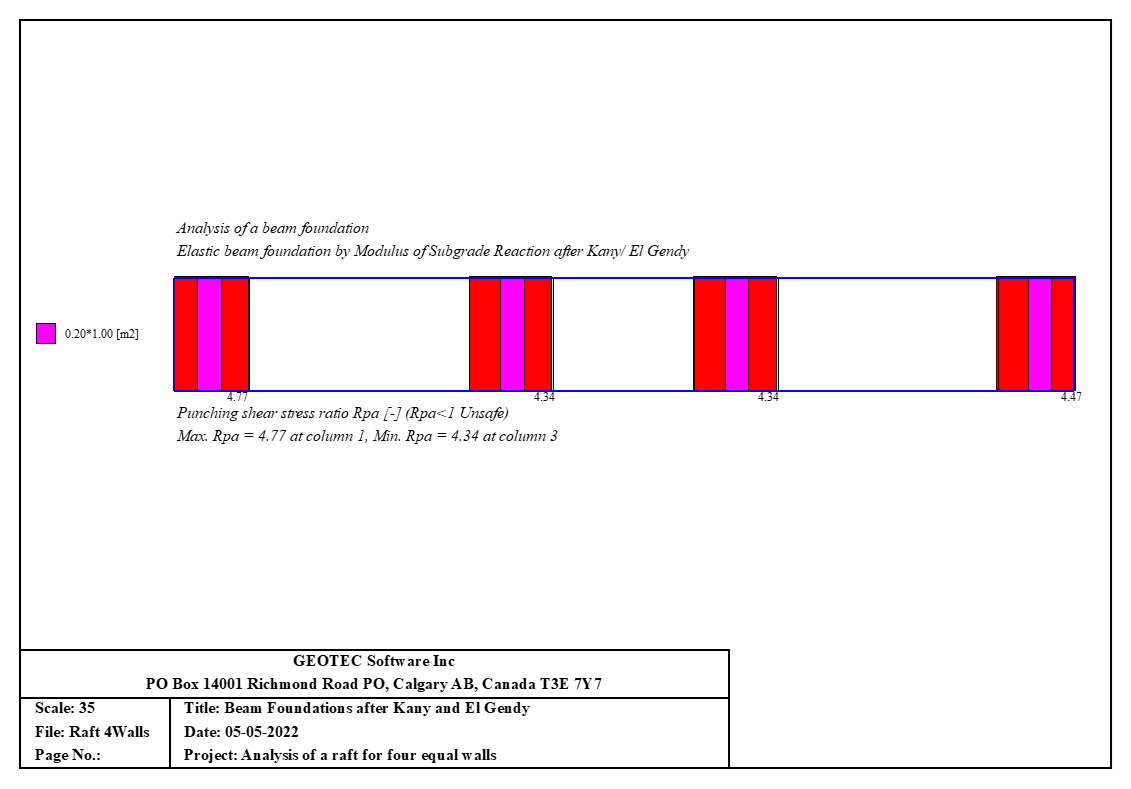The modified Cam-Clay model is a widely used constitutive model for soil mechanics that can capture the nonlinear elastic and plastic behavior of saturated clay soils under various loading conditions. The model is based on the concept of critical state soil mechanics, which assumes that there is a unique relationship between the mean effective stress, the deviatoric stress, and the specific volume of the soil at the critical state, where the soil flows as a frictional fluid without any change in stress or volume. The model uses an elliptical yield surface in the mean effective stress-deviatoric stress plane, which evolves with plastic deformation according to a hardening rule that depends on the specific volume. The model also uses an associated flow rule, which implies that the direction of plastic strain increment is normal to the yield surface. The model parameters are determined from experimental data, such as isotropic compression tests and triaxial tests, and can be related to soil properties such as initial void ratio, pre-consolidation pressure, and friction angle. The modified Cam-Clay model can reproduce some important features of clay behavior, such as compression and swelling, shear hardening and softening, and dilatancy and contractancy.